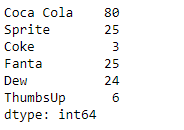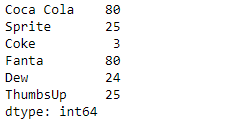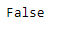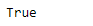Open in App
Not now

# Python | Pandas Series.equals()

• Last Updated : 13 Feb, 2019

Pandas series is a One-dimensional ndarray with axis labels. The labels need not be unique but must be a hashable type. The object supports both integer- and label-based indexing and provides a host of methods for performing operations involving the index.

Pandas` Series.equals()` function test whether two objects contain the same elements. This function allows two Series or DataFrames to be compared against each other to see if they have the same shape and elements.

Syntax: Series.equals(other)

Parameter :
other : The other Series or DataFrame to be compared with the first.

Returns : True if all elements are the same in both objects, False otherwise.

Example #1: Use `Series.equals()` function to check whether the underlying data in the two given series objects are same or not.

 `# importing pandas as pd``import` `pandas as pd`` ` `# Creating the first Series``sr1 ``=` `pd.Series([``80``, ``25``, ``3``, ``25``, ``24``, ``6``])`` ` `# Creating the second Series``sr2 ``=` `pd.Series([``80``, ``25``, ``3``, ``80``, ``24``, ``25``])`` ` `# Create the Index``index_ ``=` `[``'Coca Cola'``, ``'Sprite'``, ``'Coke'``, ``'Fanta'``, ``'Dew'``, ``'ThumbsUp'``]`` ` `# set the first series index``sr1.index ``=` `index_`` ` `# set the second series index``sr2.index ``=` `index_`` ` `# Print the first series``print``(sr1)`` ` `# Print the second series``print``(sr2)`

Output :Now we will use `Series.equals()` function to check if the underlying data in the two given series objects are same or not.

 `# check for equality``result ``=` `sr1.equals(other ``=` `sr2)`` ` `# Print the result``print``(result)`

Output :As we can see in the output, the `Series.equals()` function has returned `False` indicating the element in the two given series objects are not same.

Example #2: Use `Series.equals()` function to check whether the underlying data in the two given series objects are same or not.

 `# importing pandas as pd``import` `pandas as pd`` ` `# Creating the first Series``sr1 ``=` `pd.Series([``80``, ``25``, ``3``, ``25``, ``24``, ``6``])`` ` `# Creating the second Series``sr2 ``=` `pd.Series([``80``, ``25``, ``3``, ``25``, ``24``, ``6``])`` ` `# Create the Index``index_ ``=` `[``'Coca Cola'``, ``'Sprite'``, ``'Coke'``, ``'Fanta'``, ``'Dew'``, ``'ThumbsUp'``]`` ` `# set the first series index``sr1.index ``=` `index_`` ` `# set the second series index``sr2.index ``=` `index_`` ` `# Print the first series``print``(sr1)`` ` `# Print the second series``print``(sr2)`

Output :Now we will use `Series.equals()` function to check if the underlying data in the two given series objects are same or not.

 `# check for equality``result ``=` `sr1.equals(other ``=` `sr2)`` ` `# Print the result``print``(result)`

Output :As we can see in the output, the `Series.equals()` function has returned `True` indicating the element in the two given series objects are same.

My Personal Notes arrow_drop_up